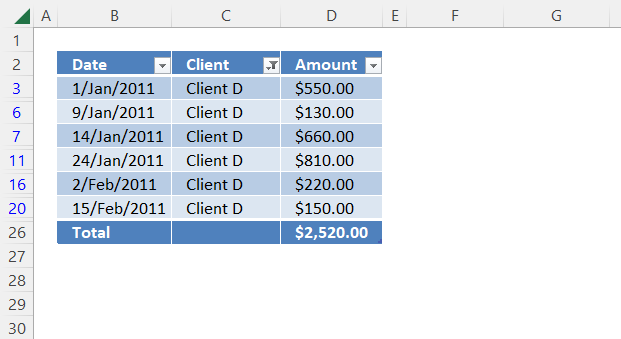Author: Oscar Cronquist Article last updated on October 14, 2022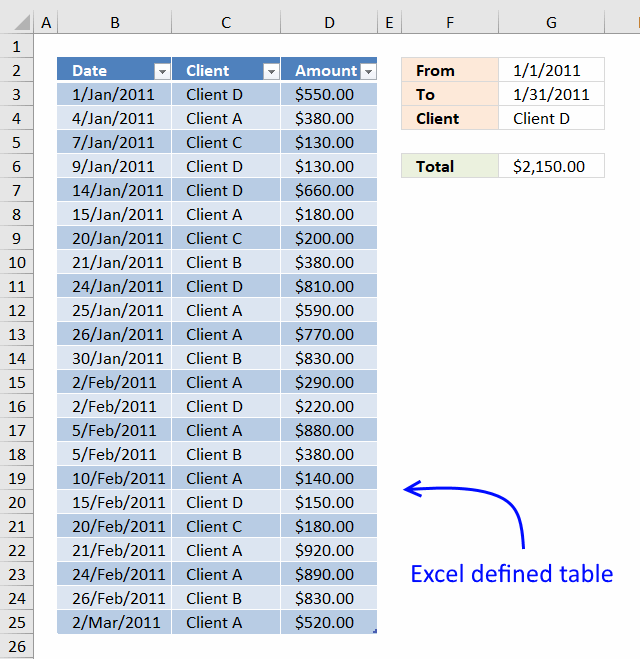LOVE this example, my issue/need is, I need to add the results. So instead of States and Names, I have to match a client name, and add all the sales totals:

clientA 10
clientA 10
clientA 10
clientB 5
clientB 5
clientB 5

So if I search for clientA, I need one cell that keeps a running total as sales are added. Lastly, a date range will need to be given, so search for sales from clientA between two dates and keep a running total...

## 1. Running totals based on criteria - formula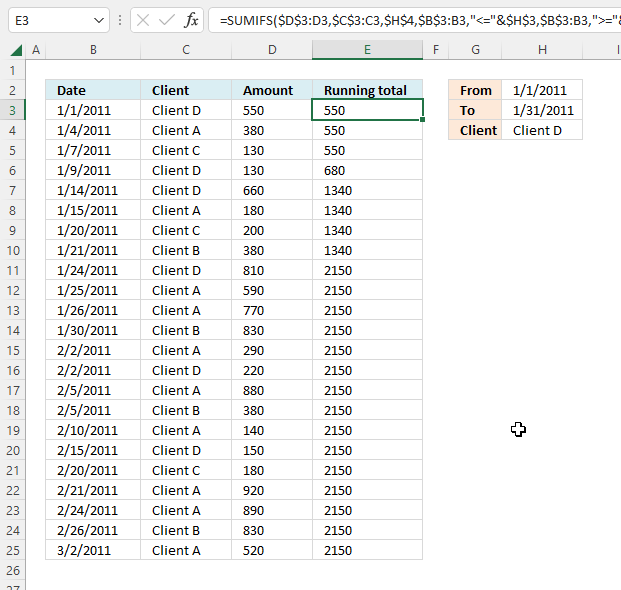The image above demonstrates a formula in cell E3 that calculates a running total based on a date range specified in cells H2, H3, and a condition specified in cell H4.

Formula in cell E3:

=SUMIFS(\$D\$3:D3,\$C\$3:C3,\$H\$4,\$B\$3:B3,"<="&\$H\$3,\$B\$3:B3,">="&\$H\$2)

### Explaining the formula in cell E3

#### Step 1 - Populate SUMIFS function arguments

The SUMIFS function adds numbers based on criteria.

Function syntax: SUMIFS(sum_range, criteria_range1, criteria1, [criteria_range2], [criteria2], ...)

sum_range - \$D\$3:D3

criteria_range1 - \$C\$3:C3

criteria1 - \$H\$4

[criteria_range2] - \$B\$3:B3

[criteria2] - "<="&\$H\$3

[criteria_range3] - \$B\$3:B3

[criteria3] - ">="&\$H\$2

Cell reference \$D\$3:D3 contains an absolute part \$D\$3 indicated by the dollar signs and a relative part D3. This makes the cell reference grow when the cell is copied to the cells below.

This is also the case with \$C\$3:C3 and \$B\$3:B3, this makes the formula include more and more cells in columns B, C, and D creating the running total effect.

The less than, greater than, and equal sign shown in "<="&\$H\$3 and ">="&\$H\$2 let you create a condition so that the date range criteria are met.

The third condition is specified in cell \$H\$4, it makes sure that the running total is only valid for "Client D".

SUMIFS(sum_range, criteria_range1, criteria1, [criteria_range2], [criteria2], [criteria_range3], [criteria3], ...)

becomes

SUMIFS(\$D\$3:D3,\$C\$3:C3,\$H\$4,\$B\$3:B3,"<="&\$H\$3,\$B\$3:B3,">="&\$H\$2)

#### Step 2 - Evaluate SUMIFS function

SUMIFS(\$D\$3:D3,\$C\$3:C3,\$H\$4,\$B\$3:B3,"<="&\$H\$3,\$B\$3:B3,">="&\$H\$2)

becomes

SUMIFS(550,"Client D","Client D",40544,"<=40574",40544,">=40544")

and returns

550.

## 2. Running totals based on criteria - formula and Excel Table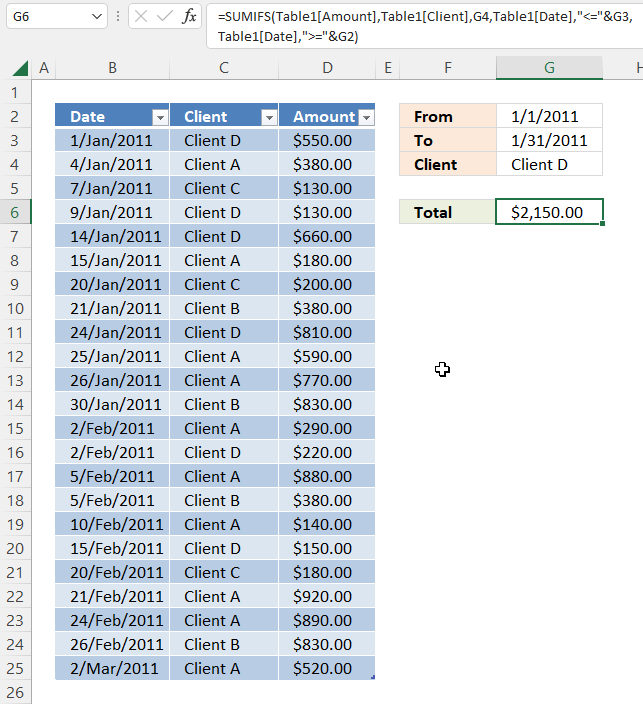I highly recommend a pivot table for this task, however, this article demonstrates a formula combined with an Excel defined table. A pivot table is lightning fast if you have lots of data to work with and is easy to learn.

The Excel Table is great for a list that keeps growing, the references to the Excel Table don't change which makes the formula easy to use. There is no need to adjust cell references, they are called structured references and are only used in Excel Tables.

This example shows how to calculate a running total using a formula based on a date range and a condition. This example is different from the one in section 1, it calculates a total for all data in the Excel Table. You can add more data to the Excel Table and the formula adjusts automatically creating a running total.

Formula in cell G6:

=SUMIFS(Table1[Amount], Table1[Client], G4, Table1[Date], "<="&G3, Table1[Date], ">="&G2)

The SUMIFS function was introduced in Excel 2007, use the SUMPRODUCT function if you have an earlier Excel version.

=SUMPRODUCT(Table1[Amount]*(Table1[Client]=G4)*(Table1[Date]<=G3)*(Table1[Date]>=G2))

### How to create an Excel defined table

1. Select a cell in the data set.
2. Press CTRL + T
3. A dialog box appears, enable checkbox if your data set has headers.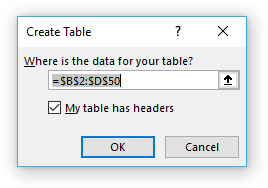4. Press with left mouse button on OK.

### Explaining the formula in cell G6

Some of the cell references in the SUMIFS function are structured references pointing to a range in an Excel defined table. Structured references adjust automatically when data is added or removed to the table, the formula will instantly return the new running total.

Structured reference -> Table1[Amount]

The SUMIFS function adds numbers based on criteria and returns the total.

SUMIFS(sum_range, criteria_range1criteria1[criteria_range2], [criteria2], ...)

#### Step 1 - First argument is sum_range

The sum_range argument is a structured reference: Table1[Amount]. This cell range contains the numbers to be added based on criteria.

#### Step 2 - Second and third argument

The first pair of arguments evaluates if cells in column Client equal value in cell G4.

 Argument Cell reference criteria_range1 Table1[Client] criteria1 G4

#### Step 3 - Fourth and fifth argument

The second pair of arguments evaluates if cells in column Date are equal or are less than value in cell G3.

 Argument Cell reference criteria_range2 Table1[Date] criteria2 "<="&G3

The less than and equal signs are concatenated to cell reference G3 using the ampersand character.

#### Step 4 - Sixth and seventh argument

The third pair of arguments evaluates if cells in column Date are equal or are larger than the value in cell G2.

 Argument Cell reference criteria_range3 Table1[Date] criteria3 ">="&G2

The larger than and equal signs are concatenated to cell reference G2 using the ampersand character.

## 3. Get the Excel file

### Get the Excel fileRunning-totals-within-date-range.xlsx

## 4. Running totals based on criteria - Excel Table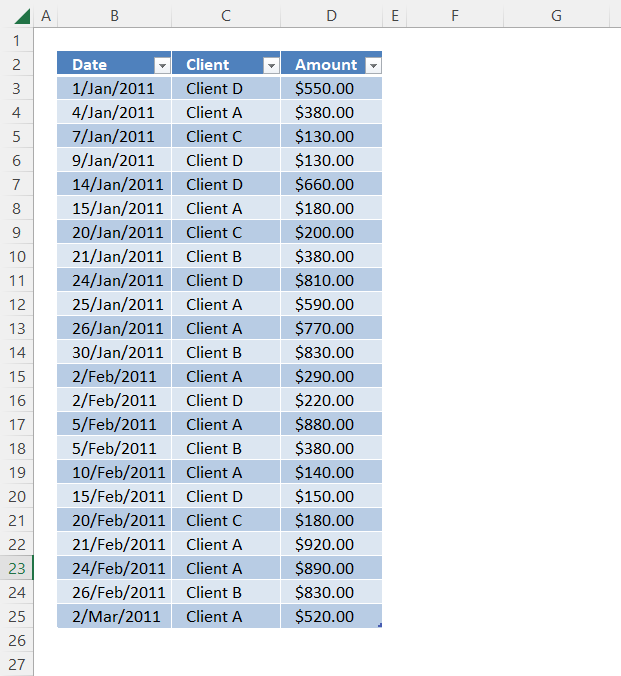This example shows how to calculate a running total using an Excel Table only based on a date range and a condition.

Excel 2007 and later versions let you add a total row below the Excel Table. Here is how to enable the total row:

1. Select any cell in the Excel Table.
2. A new tab on the ribbon appears named "Table Design", press with left mouse button on that tab to select it.
3. Press with mouse on the "Total row" check box to enable it.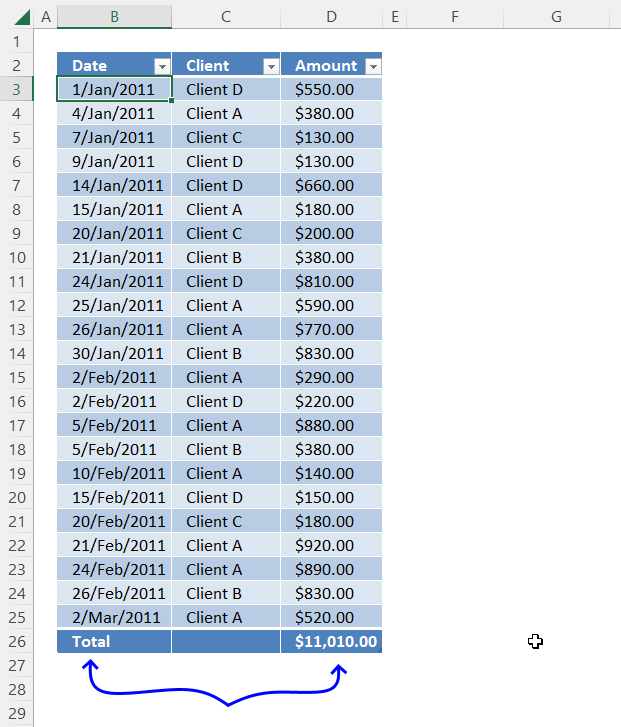The total row is now visible.

### 4.1 How to filter an Excel Table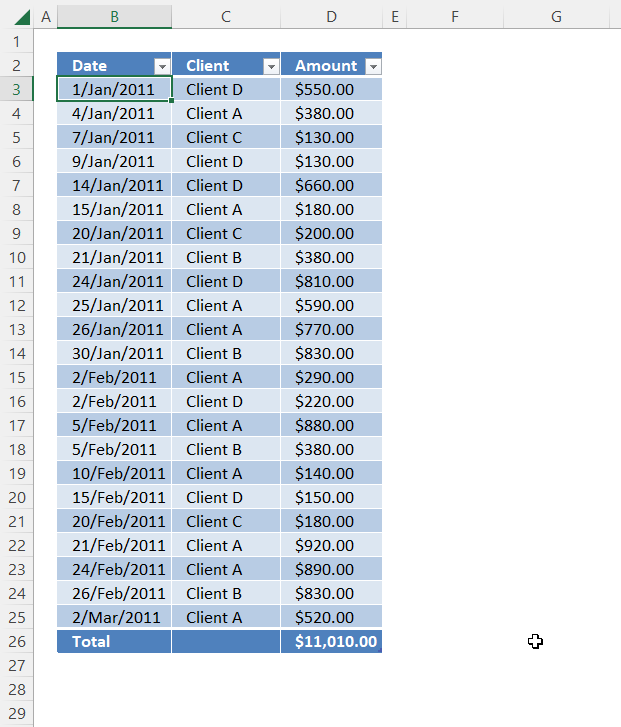The Excel Table has small buttons containing an arrow next to the column header names. Press with mouse on the button next to "Date".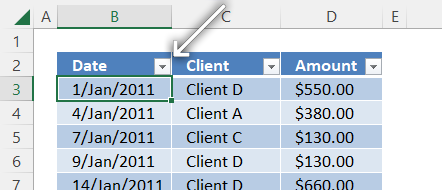A popup menu appears, deselect all checkboxes except "January".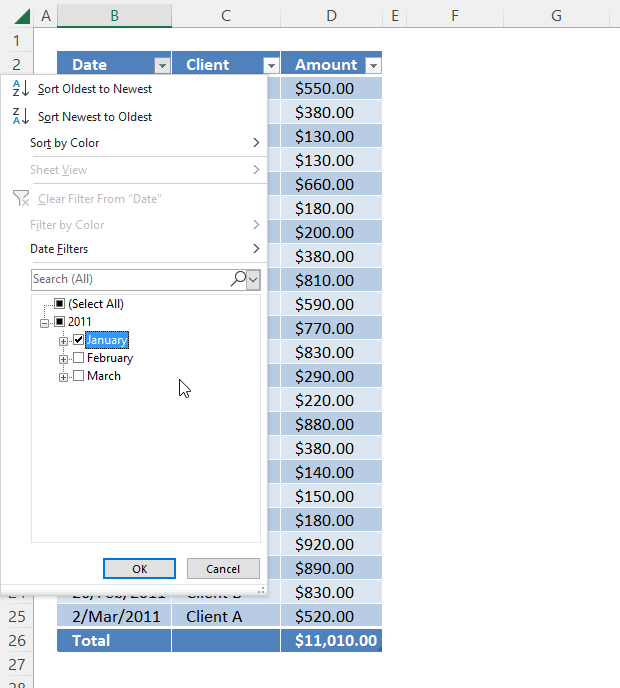Press with left mouse button on the "OK" button.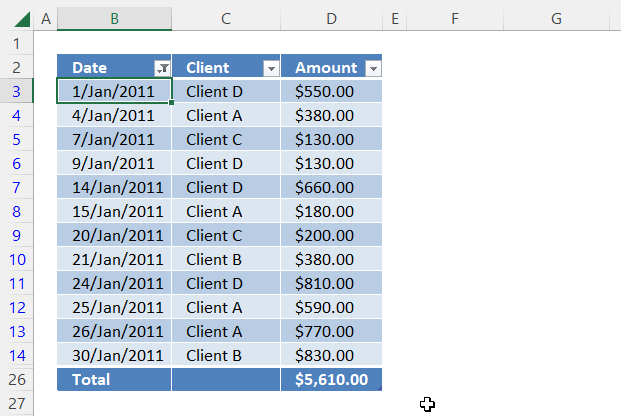Note, the button next to the column header name "Date" has changed, and the new icon tells you that a filter is present.

Press with left mouse button on the button next to the column header name "Client", and a popup menu appears.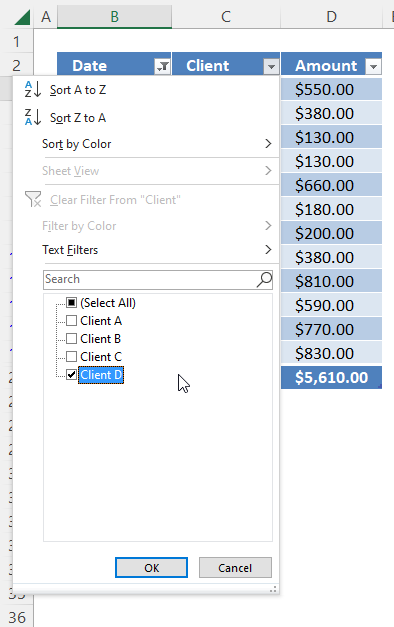Deselect all check boxes except "Client D", then press with left mouse button on the "OK" button.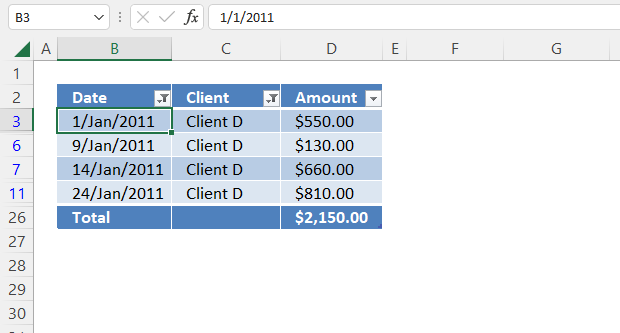### 4.2 How to clear an Excel Table filterPress with left mouse button on the button next to the column header name you want to clear, a popup menu appears.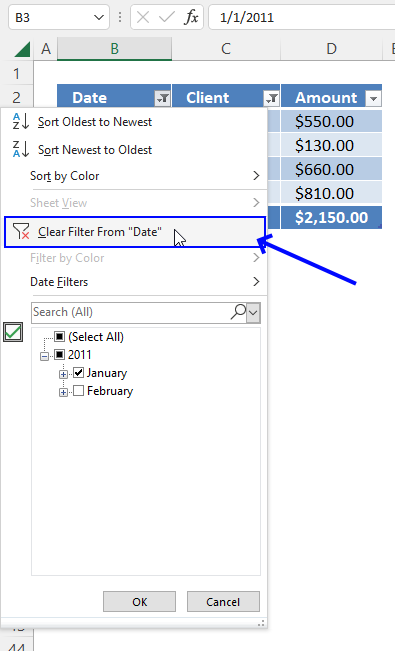Press with left mouse button on "Clear Filter From "Date".